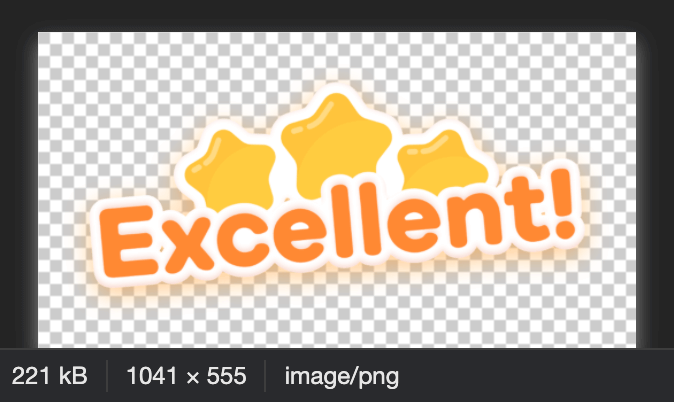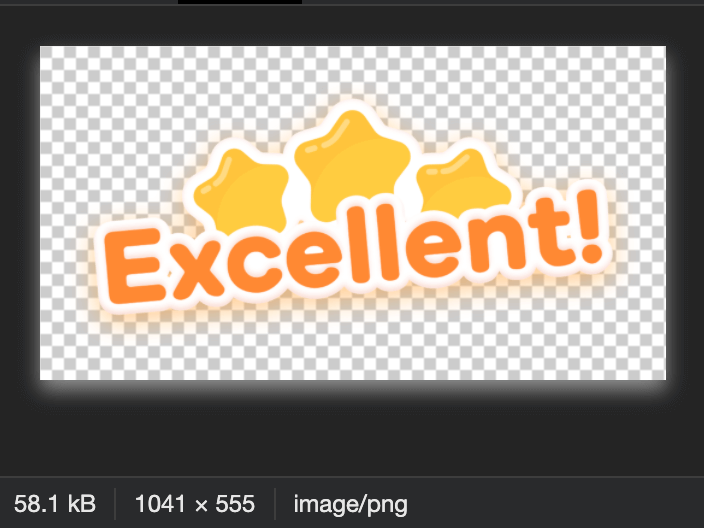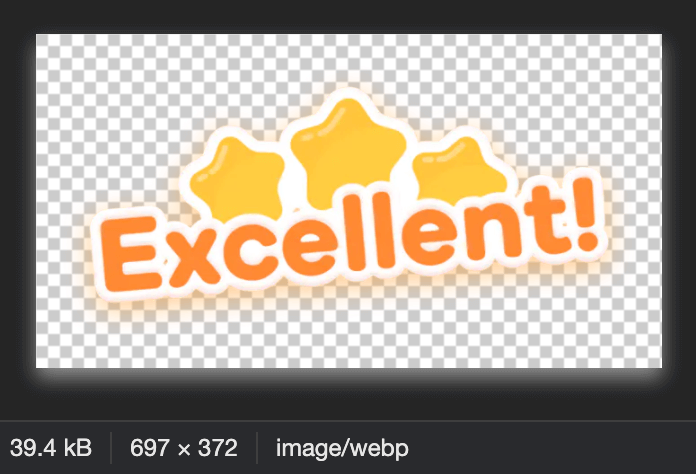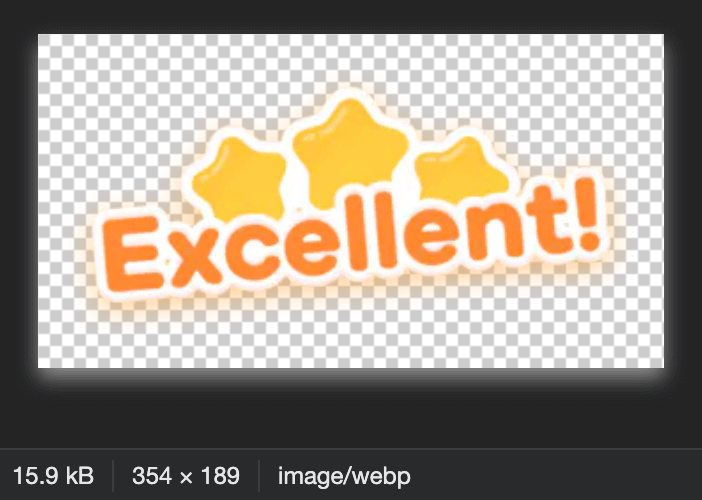# vue 项目极限优化，提升首屏访问体验

## 首屏加载视觉提速

``````<div id="app">
<div style="margin: auto">
width="200px" height="200px" viewBox="0 0 100 100" preserveAspectRatio="xMidYMid">
<g transform="translate(20 50)">
<circle cx="0" cy="0" r="6" fill="#31ca89" transform="scale(0.00244898 0.00244898)">
<animateTransform attributeName="transform" type="scale" begin="-0.375s" calcMode="spline"
keySplines="0.3 0 0.7 1;0.3 0 0.7 1" values="0;1;0" keyTimes="0;0.5;1" dur="1s" repeatCount="indefinite">
</animateTransform>
</circle>
</g>
<g transform="translate(40 50)">
<circle cx="0" cy="0" r="6" fill="#ef465d" transform="scale(0.197344 0.197344)">
<animateTransform attributeName="transform" type="scale" begin="-0.25s" calcMode="spline"
keySplines="0.3 0 0.7 1;0.3 0 0.7 1" values="0;1;0" keyTimes="0;0.5;1" dur="1s" repeatCount="indefinite">
</animateTransform>
</circle>
</g>
<g transform="translate(60 50)">
<circle cx="0" cy="0" r="6" fill="#ff9936" transform="scale(0.537416 0.537416)">
<animateTransform attributeName="transform" type="scale" begin="-0.125s" calcMode="spline"
keySplines="0.3 0 0.7 1;0.3 0 0.7 1" values="0;1;0" keyTimes="0;0.5;1" dur="1s" repeatCount="indefinite">
</animateTransform>
</circle>
</g>
<g transform="translate(80 50)">
<circle cx="0" cy="0" r="6" fill="#ffe700" transform="scale(0.862077 0.862077)">
<animateTransform attributeName="transform" type="scale" begin="0s" calcMode="spline"
keySplines="0.3 0 0.7 1;0.3 0 0.7 1" values="0;1;0" keyTimes="0;0.5;1" dur="1s" repeatCount="indefinite">
</animateTransform>
</circle>
</g>
</svg>
</div>
</div>
</div>``````

## 资源加载可视化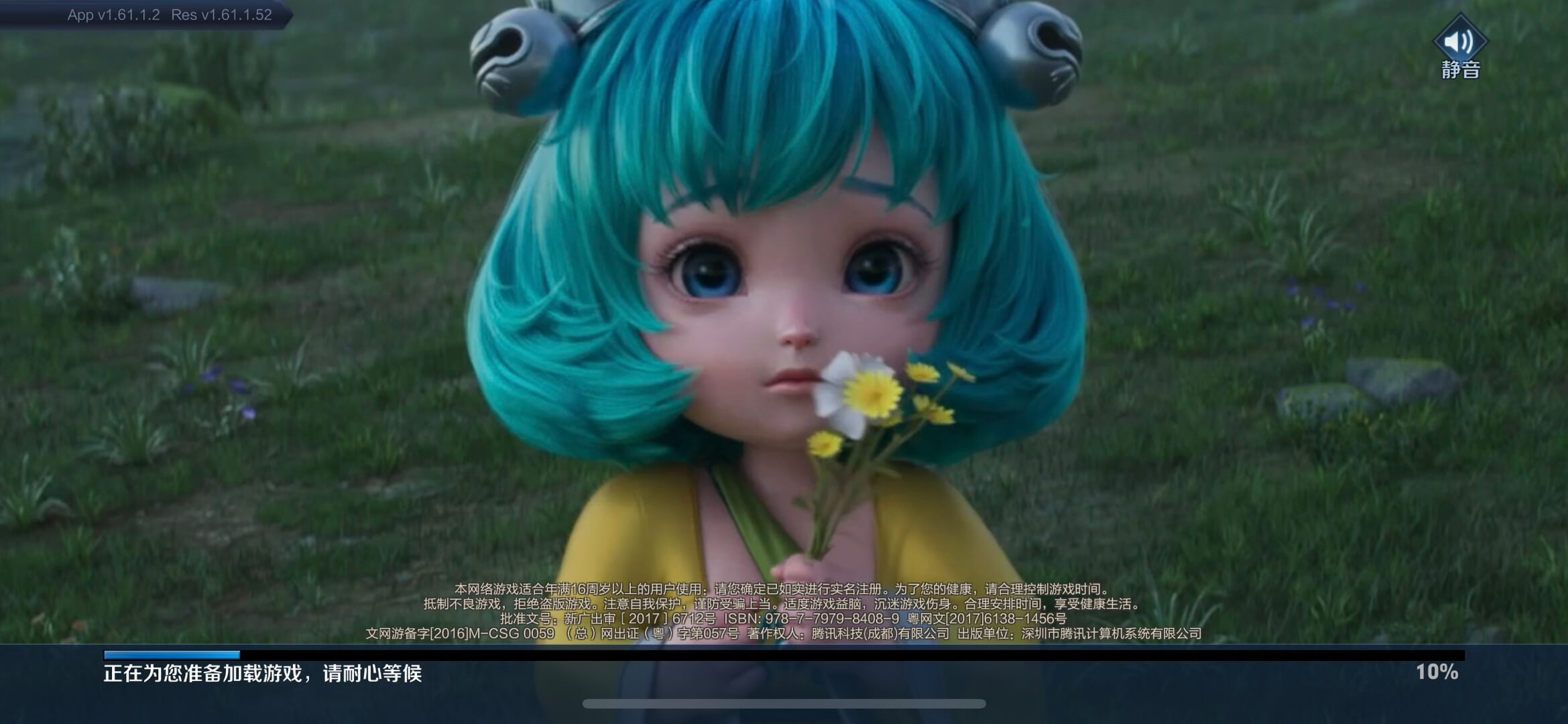``````<template>
<div class="progress-bar">
<div class="progress" :style="{ width: `\${currentProgress}%` }"></div>
</div>
<slot v-if="currentProgress === 100"></slot>
</section>
</template>``````
``````const lottiejs = () => {
return import(/* webpackChunkName: "lottie" */ 'lottie-web')
}
const animate = () => {
return import(/* webpackChunkName: "animate" */ '@/assets/animate.json')
}

const loader = [lottiejs(), animate()].map((item) => {
return item
.then((data) => {
return data
})
.catch((err) => {
console.error(err.message)
})
})
})
const timer = setInterval(() => {
if (this.currentProgress < 100) {
if (this.currentProgress > 100) this.currentProgress = 100
} else {
clearInterval(timer)
}
}, 300)``````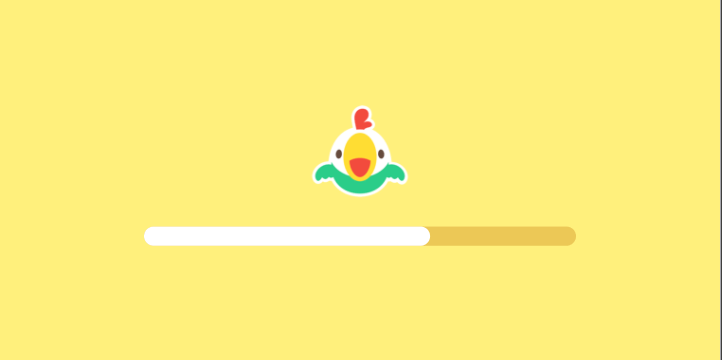## 图片最优化和预加载

### 图片预加载

``````function loadImage(url) {
return new Promise((resolve, reject) => {
// 利用新建一个图片实例而不渲染实现图片预加载
const image = new Image()
image.onerror = () => reject(new Error(`Image preload error: \${url}`))
image.src = url
})
}``````

``````// thread，表示每一批的资源数量
})
})
}``````

### 图片最优化

``````let checkedWebp = false
function slimImages(images) {
if (checkedWebp) return images
// checkWebpFeature 函数的实现，你可以参考网上的方案，这里由于篇幅原因不再展开
return checkWebpFeature('alpha')
.then((supportWebp) => {
if (supportWebp) {
_.entries(images).forEach(([key, val]) => {
images[key] = `\${val}?imageMogr2/thumbnail/!\${Math.ceil((window.devicePixelRatio / 3) * 100) || 100}p/format/webp`
})
}
return images
})
.finally(() => {
checkedWebp = true
})
}``````

1、不同分辨率的设备使用对应尺寸的图片 `Math.ceil((window.devicePixelRatio / 3) * 100)`，七牛云的 `thumbnail/!100p` 指令可以生成对应比例的图片，其中 100p 代表原始大小。
2、使用了 webp 格式，webp 是 Google 早期推出的一种高压缩的图片格式。这种图片格式据说曾帮助淘宝每年节省好几亿的流量费，Amazing！

#### 总结